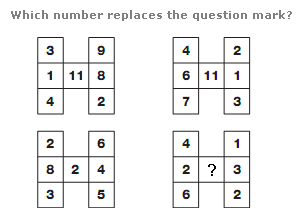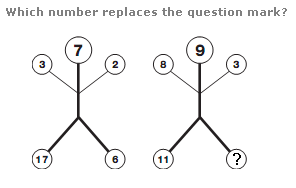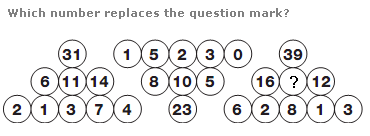# Puzzles - Number puzzles

### Exercise :: Number puzzlesAnswer : 6 Explanation : In each diagram, the central value equals the difference between the sums of the numbers in the left hand column and the sums of the numbers in the right hand column.Answer : 14 Explanation : In each diagram, the number at the top equals the average of the four smaller numbers.Answer : 11 Explanation : In each triangular group of circles, start with the longest row and add together 3 adjacent values and put this sum in the circle directly above or below the centre of the 3 values. Continue this sequence towards the apex of each triangle.